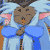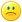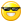## Welcome to the Second Life Forums Archive

These forums are CLOSED. Please visit the new forums HERE

# integer <-> binary (string)

Strife Onizuka
MoonchildJoin date: 3 Mar 2004
Posts: 5,887
04-21-2004 11:09
CODE
`string integer2binary(integer a){    integer c;    string d;    for (c=1;c && (a<0 || (c<=a && a>0) || (a==0 && c==1));c*=2)        d+=(string)((a&c)!=0);    return d;}integer binary2integer(string a){    integer b;    integer c;    integer d;    for (c=1;b<llStringLength(a) && c;c*=2)        d=d|(c*(llGetSubString(a,b,b++)=="1"));    return d;}`

edit:
fix for infinate loop integer2binary (didn't show up in my testing).
_____________________
Truth is a river that is always splitting up into arms that reunite. Islanded between the arms, the inhabitants argue for a lifetime as to which is the main river.
- Cyril Connolly

Without the political will to find common ground, the continual friction of tactic and counter tactic, only creates suspicion and hatred and vengeance, and perpetuates the cycle of violence.
- James Nachtwey
Strife Onizuka
MoonchildJoin date: 3 Mar 2004
Posts: 5,887
04-22-2004 00:49
Wrote these to help with bitfeild manipulations as it is hard when tired to think in bits. binary2integer was an afterthought.

"integer c" is used to represent every bit by shifting it the bit over; multiplying it by 2 does the shift (*curses LSL for not having bitshifts*).

CODE
`(binary)10000000 * 01000000 = 01000000 (2)01000000 * 01000000 = 00100000 (4)00100000 * 01000000 = 00010000 (8)00010000 * 01000000 = 00001000 (16)00001000 * 01000000 = 00000100 (32)00000100 * 01000000 = 00000010 (64)00000010 * 01000000 = 00000001 (-128)00000001 * 01000000 = 00000000 (0)this was only an 8 bit representation. LSL is 32.the last bit is used to represent the negative bit.`

The code depends on integer arithmatic overflowing into the negative. ie (2 * 1073741824 = -214748364and (2 * -2147483648 = 0) That is why we do a check for (c && a<0) as the negative bit is last. (If LSL ever goes to long long (64bit integers) then this code will still work as the overflow will just be at a different point.) When a>0 all we need to do is check which is bigger c or or a and if a is bigger than we contiue. finaly if we check if a==0 so we can run the loop once as to return "0".

Then for checking the value of the bit in that possition we just and c & a, which will return c if there is a 1 and 0 if nothing. Since compairing it to zero is the fastest way for checking that is what we do.

-----

binary2integer we do something similar. We still have c and it increments in the same way. We read the string 1 letter at a time and see if it "1" this test returns a 1 or a 0. We multiply the result of the test by c then Or it to d (same effect as adding it but a bit faster)

-----

Issues:
Since LSL is 32 bit if you try to feed a longer binary number to binary2integer the extra bits will be lost as C will overflow into 0. We do a check for this as it's just a waste of CPU time to continue.
binary2integer only does a check for "1" so this function will try and parse any string and return a number.

-----

Optimization:
If your worried about CPU time then you should use this version of integer2binary
CODE
`string integer2binary(integer a){    integer c;    string d;    if (a<0)        for (c=1;c;c*=2)            d+=(string)((a&c)!=0);    else if (a)        for (c=1;c<=a && c;c*=2)            d+=(string)((a&c)!=0);    else        d="0";    return d;}`
_____________________
Truth is a river that is always splitting up into arms that reunite. Islanded between the arms, the inhabitants argue for a lifetime as to which is the main river.
- Cyril Connolly

Without the political will to find common ground, the continual friction of tactic and counter tactic, only creates suspicion and hatred and vengeance, and perpetuates the cycle of violence.
- James Nachtwey